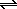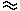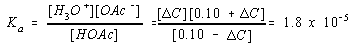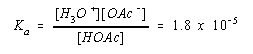Mixtures and Buffers

Mixtures and Buffers

Mixtures of Acids and Bases

What would happen if we added enough sodium acetate to an acetic acid solution so that the solution is simultaneously 0.10 M in both HOAc and NaOAc?

 0.10 M HOAc: [H3O+] = 1.3 x 10-3 M pH = 2.9 0.10 M NaOAc: [OH-] = 7.5 x 10-6 M pH = 8.9

The first step toward answering this question is recognizing that there are two sources of the OAc- ion in this solution. Acetic acid, of course, dissociates to give the H3O+ and OAc- ions.

HOAc(aq) + H2O(l)H3O+(aq) + OAc-(aq)

Sodium acetate, however, also dissociates in water to give the OAc- ion.

 H2O NaOAc(s)Na+(aq) + OAc-(aq)

These reactions share a common ion: the OAc- ion. LeChatelier's principle predicts that adding NaOAc to an HOAc should shift the equilibrium between HOAc and the H3O+ and OAc- ions to the left. Thus, adding NaOAc reduces the extent to which HOAc dissociates. This mixture is therefore less acidic than 0.10 M HOAc, by itself.

We can quantitate this discussion by setting up the problem as follows.

 HOAc(aq) + H2O(l)H3O+(aq) + OAc-(aq) Ka = 1.8 x 10-5 Initial: 0.10 M0 0.10 M Equilibrium: ? ? ?

We don't have any basis for predicting whether the dissociation of water can be ignored in this calculation, so let's make this assumption and check its validity later.

If most of the H3O+ ion concentration at equilibrium comes from the dissociation of the acetic acid, the reaction has to shift to the right to reach equilibrium.

 HOAc(aq) + H2O(l)H3O+(aq) + OAc-(aq) Ka = 1.8 x 10-5 Initial: 0.10 M0 0.10 M Equilibrium: 0.10 -CC 0.10 +C

Substituting this information into the Ka expression gives the following result.By now, the next step should be obvious: We assume thatC is small compared with the initial concentrations of HOAc and OAc-.We then solve the approximate equation this assumption generates.C1.8 x 10-5

Are our two assumptions legitimate? IsCsmall enough compared with 0.10 to be ignored? IsC large enough so that the dissociation of water can be ignored? The answer to both questions is yes. We can therefore use this value ofC to calculate the H3O+ ion concentration at equilibrium and the pH of the solution.

pH = - log [1.8 x 10-5] = 4.74

This solution is therefore acidic, but less acidic than HOAc by itself.Buffers

Mixtures of a weak acid and its conjugate base, such as HOAc and the OAc- ion, are called buffers. The term buffer usually means "to lessen or absorb shock." These solutions are buffers because they lessen or absorb the drastic change in pH that occurs when small amounts of acids or bases are added to water. Adding a single drop of 2 M hydrochloric acid to 100 mL of pure water changes the pH from 7 to 3.

 Practice Problem 6:Let's see what happens when we add a drop of 2 M HCl to a buffer solution. Calculate the effect of adding one drop of 2 M HCl to 100 mL of a buffer solution that is 0.100 M in both acetic acid and sodium acetate. Click here to check your answer to Practice Problem 6 Click here to see a solution to Practice Problem 6

Although a single drop of 2 M HCl reduces the pH of 100 mL of water by 4 pH units (from 7 to 3), there is no change in the pH of the buffer when a drop of 2 M HCl is added to 100 mL of this buffer solution. Thus, the pH of the buffer solution is truly "buffered" against the effect of small amounts of acid or base.

Buffers can be made from a weak acid and its conjugate base, such as acetic acid and a salt of the acetate ion.

 HOAc(aq) + H2O(l)H3O+(aq) + OAc-(aq) weak acid conjugate base

Buffers can also be made from a weak base and its conjugate acid, such as ammonia and a salt of the ammonium ion.

 NH4+(aq) + H2O(l)H3O+(aq) + NH3(aq) conjugate acid weak base

There is an important difference between these buffers. Mixtures of HOAc and the OAc- ion form an acidic buffer, with a pH below 7. Mixtures of NH3 and the NH4+ ion form a basic buffer, with a pH above 7.

We can predict whether a buffer will be acidic or basic by comparing the values of Ka and Kb for the conjugate acid-base pair. Ka for acetic acid is significantly larger than Kb for the acetate ion. We therefore expect mixtures of this conjugate acid-base pair to be acidic.

 HOAc: Ka = 1.8 x 10-5 OAc-: Kb = 5.6 x 10-10

Kb for ammonia, on the other hand, is much larger than Ka for the ammonium ion. Mixtures of this acid-base pair are therefore basic.

 NH3: Kb = 1.8 x 10-5 NH4+: Ka = 5.6 x 10-10

In general, if Ka for the acid is larger than 1 x 10-7, the buffer will be acidic. If Kb is larger than 1 x 10-7, the buffer is basic.Buffer Capacity and pH Titration Curves

The most important property of a buffer is its ability to resist changes in pH when small quantities of acid or base are added to the solution. We can develop a model for the capacity of a buffer to absorb acid or base by looking at how the buffer resists changes in pH. Consider an HOAc/OAc- buffer, for example.Rearranging the Ka expression for this buffer gives the following equation.According to this equation, the H3O+ ion concentration, and therefore the pH of the solution, will remain essentially constant as long as the ratio of the concentrations of HOAc and the OAc- ion is more or less constant.

Suppose we start with a solution that contains equal amounts of HOAc and the OAc- ion. When we add small amounts of acid to this solution, some of the acetate ions are converted into acetic acid.

OAc-(aq) + H3O+(aq)HOAc(aq) + H2O(l)

But the ratio of these concentrations doesn't change by much, so the pH stays the same. When we add small amounts of base to the solution, some of the acetic acid is converted into acetate ions.

HOAc(aq) + OH-(aq)OAc-(aq) + H2O(l)

But, once again, the ratio of these concentrations stays more or less the same, and so does the pH.

As long as the concentrations of HOAc and the OAc- ion in the buffer are larger than the amount of acid or base added to the solution, the pH remains constant. The table below compares the effects of adding different amounts of hydrochloric acid to water and a pair of buffer solutions. The first column gives the number of moles of HCl added per liter. The second column shows the effect of this much acid on the pH of water. The third column shows the effect on a buffer solution that contains 0.10 M HOAc and 0.10 M NaOAc. The fourth column shows the results of adding the acid to a buffer that contains twice as much acetic acid and twice as much sodium acetate.

The Effect of Adding Hydrochloric Acid to Water and Two Buffer Solutions

 Mol HCl per L of solution pH of water pH of Buffer 1 (0.10 M HOAc/ 0.10 M OAc-) pH of Buffer 2 (0.20 M HOAc/ 0.20 M OAc-) 0 7 4.74 4.74 0.000001 6 4.74 4.74 0.00001 5 4.74 4.74 0.0001 4 4.74 4.74 0.001 3 4.74 4.74 0.01 2 4.66 4.70 0.1 1 2.72 4.27

Even when as much as 0.01 moles per liter of acid is added to the first buffer, there is very little change in the pH of the buffer solution, as shown in the figure below.By definition, the buffer capacity of a buffer is the amount of acid or base that can be added before the pH of the solution changes significantly. Note that the capacity of the second buffer solution in the table above is even greater than the first. This buffer protects against quantities of acid or base as large as 0.1 moles per liter.

Buffers and buffering capacity have a lot to do with the shape of the titration curve in the figure below, which shows the pH of a solution of acetic acid as it is titrated with a strong base, sodium hydroxide. Four points (A, B, C, and D) on this curve will be discussed in some detail.Point A represents the pH at the start of the titration. The pH at this point is 2.9, the pH of an 0.10 M HOAc solution. As NaOH is added to this solution, some of the acid is converted to its conjugate base, the OAc- ion.

HOAc(aq) + OH-(aq)OAc-(aq) + H2O(l)

Point B is the point at which exactly half of the HOAc molecules have been converted to OAc- ions. At this point, the concentration of the HOAc molecules is equal to the concentration of the OAc- ions. Because the ratio of the HOAc and OAc- concentrations is 1:1, the concentration of the H3O+ ion at Point B is equal to the value of Ka for the acid.

 Point B: [H3O+] = Ka

This provides us with a way of measuring Ka for an acid. We can titrate a sample of the acid with a strong base, plot the titration curve, and then find the point along this curve at which exactly half of the acid has been consumed. The H3O+ ion concentration at this point will be equal to the value of Ka for the acid.

Point C is the equivalence point for the titrationthe point at which enough base has been added to the solution to consume the acid present at the start of the titration. The goal of any titration is to find the equivalence point. What is actually observed is the endpoint of the titrationPoint Dthe point at which the indicator changes color.

Every effort is made to bring the endpoint as close as possible to the equivalence point of a titration. Suppose that phenolphthalein is used as the indicator for this titration, for example. Phenolphthalein turns from colorless to pink as the pH of the solution changes from 8 to 10. If we wait until the phenolphthalein permanently turns pink, the endpoint will fall beyond the equivalence point for the titration. So we try to find the point at which adding one drop of base turns the entire solution to a pink color that fades in about 10 seconds while the solution is stirred. In theory, this is one drop before the endpoint of the titration and therefore closer to the equivalence point.

Note the shape of the pH titration curve in the figure above. The pH rises rapidly at first because we are adding a strong base to a weak acid and the base neutralizes some of the acid. The curve then levels off, and the pH remains more or less constant as we add base because some of the HOAc present initially is converted into OAc- ions to form a buffer solution. The pH of this buffer solution stays relatively constant until most of the acid has been converted to its conjugate base. At that point the pH rises rapidly because essentially all of the HOAc in the system has been converted into OAc- ions and the buffer is exhausted. The pH then gradually levels off as the solution begins to look like a 0.10 M NaOH solution.

The titration curve for a weak base, such as ammonia, titrated with a strong acid, such as hydrochloric acid, would be analogous to the curve in the figure above. The principal difference is fact that the pH value is high at first and decreases as acid is added.>